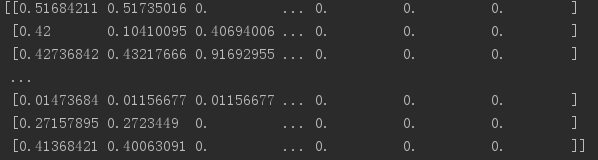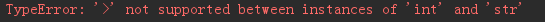• ## C/C++ int数组初始化

万次阅读 2019-01-19 14:21:02
静态数组 int array; //定义了数组array，但并未对数组初始化； 静态数组 int array = {0}; //定义了数组array，并将数组元素全部初始化为0； 静态数组 int array = {1}; //定义了数组array，并将...
一、一维数组
C/C++初始化
静态数组 int array; //定义了数组array，但并未对数组初始化；静态数组 int array = {0}; //定义了数组array，并将数组元素全部初始化为0；静态数组 int array = {1}; //定义了数组array，并将数组第一个元素初始化为1，后面99个元素初始化为0；静态数组 int array = {4,5}; //定义数组array，并初始化前两个元素为4,5，后面剩余元素初始化为0；
C++动态数组初始
动态数组 int *p_array = new int; delete []p_array; //分配了长度为100的数组array，并全部初始化为0；动态数组 int *p_array = new int{3,5}; delete []p_array; //前两个元素初始化为3和5，后面的原始初始化为0；
曾经我想将int数组元素全部初始化为1，我以为下方的写法没有问题：

int a = { 1 }; // 曾经我想全部初始化为１

事实却是，只有数组的第一个元素被初始化为１，其他全为０；
数组初始化列表中的元素个数小于指定的数组长度时，不足的元素补以默认值。
对应基本类型int来说，就是补0，再看一下非基本类型的数组：
string a = { "foo" };

有了上面的规则，就很容易知道其实相当于：
string a = { "foo", "", "", "", "" };

即后面４个元素调用了string的默认构造函数进行的初始化，而第一个则调用的string::string(const char*)进行的初始化。
还有一个区别：
int a;
string a;

如果不明确指出初始化列表，那么基本类型是不会被初始化的（除全局变量和静态变量外），所有的内存都是“脏的”；而类类型则会为每个元素调用默认构造函数进行初始化。

注意，在C++11中中间的赋值号可以省略，即 int a{1};并且，如果初始化列表为空，如 int a{}，那将初始化所有元素为默认值，即与 int a{0}; 等级

二、二维数组
C/C++初始化
静态 int array; //定义数组，并未初始化(脏数据)静态 int array = {{1},{2,2},{3,3,3}}; //数组初始化了array及array[0,1]及array[0,1,2],剩余元素初始化为0；
C++动态数组初始
动态 int (*array)[n] = new int[m][n]; delete []array; //默认初始化为0；动态 int *array = new int[m]; for(i) array[i] = new int[n]; for(i) delete []array[i]; delete []array; //多次析构，默认初始化为0；动态 int *array = new int[m][n]; delete []array; //数组按行存储，默认初始化为0
三、三位数组
C++动态数组初始 int *array = new int[m]; //只有第一维可以是变量，其他维数必须是常量，否则会报错 delete []array; //必须进行内存是否，否则内存将泄漏；
四、数组作为参数形式传递
一维数组传递
void func(int *array);void func(int array[]); //真实使用时，一般还需要给数组大小
二维数组传递
void func(int **array);void func(int (*array)[n]);
数组名作为函数形参时，在函数体内，其失去了本身的内涵，仅仅只是一个指针，而且在其失去其内涵的同时，它还失去了其常量特性，可以作自增、自减等操作，可以被修改。
五、数组重新置0
char数组
char a;
memset(a,0,sizeof(a));		//将每个元素设置为0
memset(a,1,sizeof(a));		//将每个元素设置为1
memset(a,'a',sizeof(a));	//将每个元素设置为a,‘a’ = 97 (ASCII码)
也就是说第二个元素要在ASCII码范围内(0~127)。

int数组
int a;
memset(a,0,sizeof(a));	//将每个字节设置为0，此时每个int元素正好也为0
memset(a,1,sizeof(a));	//将每个字节设置为1，此时每个int元素为16843009 = 0x1010101
// 0x1010101 = 1000000010000000100000001(二进制)

int数组示例
#include <stdio.h>
#include <string.h>

int main ()
{
int array;

memset(array,0,sizeof(array));
for(int i=0; i<10; i++)
{
printf("%d\n",array[i]);//输出0
}

memset(array,1,sizeof(array));
for(int i=0; i<10; i++)
{
printf("%d\n",array[i]);//输出16843009
}

return(0);
}

memset原型
声明：
void *memset(void *str, int c, size_t n)

将str中前n个字节用 c 替换并返回 str。 其实这里面的ch就是ascii为ch的字符； 将s所指向的某一块内存中的前n个 字节的内容全部设置为ch指定的ASCII值；
memset()的功能是对str的每一个字节进行ASCII赋值，int占四个字节，所以对int数组进行非0赋值或初始化时不能用memset()函数，只能循环处理/定义时初始化；
参考资料： https://www.cnblogs.com/SarahZhang0104/p/5749680.html
展开全文• 1、int数组其实初始化的时候默认就是全部为0 int a; int a = {0}; 以上2种写法其实都可以 注意：int a = {0};这种方法如果想把整形数组a都初始化为1就不能用了 比如int a ...
1、int数组其实初始化的时候默认就是全部为0

int a;
int a = {0};

以上2种写法其实都可以

注意：int a = {0};这种方法如果想把整形数组a都初始化为1就不能用了 比如int a = {1};//数组其实是被初始化成1,0,0,0,0   2、int数组重置为0

如果是在定义以后想全部赋为0，用memset()函数是比较快的。

int a[SIZE];
memset(a, 0, sizeof(a));
memset(a, 0, sizeof(int)*1000);//这里的1000是数组大小，需要多少替换下就可以了。

注意：memset在头文件string.h里
展开全文c c++ c语言
• ## vba数组写法

万次阅读 2008-08-28 16:44:00
读山菊花的一串串红辣椒vba数组写法2008-01-13 19:14首先 感谢山前辈的无私的奉献，惊叹山老师的技艺的高深，和娓娓道来的举例说明------------------------------红绳子 = Array("辣1", "辣2", "辣3", "辣4", "辣5...

读山菊花的一串串红辣椒vba数组写法

2008-01-13 19:14

首先 感谢山前辈的无私的奉献，惊叹山老师的技艺的高深，和娓娓道来的举例说明
------------------------------
红绳子 = Array("辣1", "辣2", "辣3", "辣4", "辣5", "辣6", "辣7", "辣8", "辣9", "辣10")    cName = Array("林思明", "曾玉婷", "曾国文", "林伟权", "林兴发", "刘卓怀", "曾仙婷", "林平")    cj = Array(99, 85, 92.5, 70, 78.5, 65, 84, 100)
-----------------------------
Private Sub CommandButton1_Click() '点击按钮C，运行本程序    cname = Array("林思明", "曾玉婷", "曾国文", "林伟权", "林兴发", "刘卓怀", "曾仙婷", "林平")        '把数组元素写入工作表c4单元格向右8个单元格内    Range("c4:j4") = cname    '或者    'Range("c4").Resize(1, 8) = cname    End Sub
Private Sub CommandButton2_Click() '点击按钮B，运行本程序    cname = Array("林思明", "曾玉婷", "曾国文", "林伟权", "林兴发", "刘卓怀", "曾仙婷", "林平")    Range("d8:d15") = WorksheetFunction.Transpose(cname)    '或者    'Range("d8").Resize(8, 1) = WorksheetFunction.Transpose(cname)End Sub
--------------------------------------
Private Sub CommandButton1_Click() '点击按钮，运行本程序    '建立数组    cname = Array("林思明", "曾玉婷", "曾国文", "林伟权", "林兴发", "刘卓怀", "曾仙婷", "林平")    '循环    For i = 1 To 8 'i是从1到8的变量                Range("a" & i) = cname(i)                '相当于：range("a1")=cname(1)，range("a2")=cname(2)……            NextEnd Sub
----------------------------------------------
Private Sub CommandButton1_Click()    xm = Split(Range("a1"), ",")    r = UBound(xm)    Range("b1:b" & r + 1) = WorksheetFunction.Transpose(xm)        MsgBox "数组最大下标是 " & r & Chr(13) _    & "数组共有 " & r + 1 & " 个元素        ", 64, "信息"End Sub
-------------------------------
Private Sub Worksheet_Change(ByVal Target As Range) '修改单元格的值时运行本程序    If Target.Address = "$D$10" Then '如果修改D10单元格的值        cname = Array("林思明", "曾玉婷", "曾国文", "林伟权", "林兴发", "刘卓怀", "曾仙婷", "林平")        Range("d11") = cname(Target) 'Target表示D10单元格的值    End IfEnd Sub
数据保存到数组   arr = Sheet2.Range("a1:e83")把数组内容写入到工作表 Range("a1:e83") = arr '光标定位到这一行，按F9设置断点。
Sub 声明数组()    Dim MyArray(10) '在过程中间声明一个数组，过程结束，本数组将被释放。    MyArray(1) = 100 '给数组赋值    MyArray(5) = 90MyArray是数组名称；10是数组最大下标。当Option Base 0的模式下，数组的序号(索引值)从0到10，表示数组有11个元素，当Option Base 1的模式下，数组的索引值从1到10，该语句创建的数组共有10个元素--------------------
Dim Data(10,5) ’声明一个二维数组    Data (1,1) = “A001” '给数组赋值Data (1,2) = 90    Data (3,1) = “A003” Data (3,2) = 80Data是一个二维数组。二维数组的两维，我们通常用“行、列”称呼它，如Data，最大行号是10，最大列号是5，在Option Base 1 模式下，表示是一个10行5列共50个元素的数组空间。在Option Base 0 模式下，表示一个11行6列共66个元素的数组空间。二维数组是使用频率最高的数组形式，因为我们的工作表也是二维的。用工作表来领会二维数组，将会更加直观：Data(1,1)相当于Cells(1,1)，表示第1行，第1列；Data(5,3)相当于Cells(5,3)，表示第5行，第3列。
-------------------
Dim x(3, 30, 3)    x(1, 1, 1) = "1班" '给数组赋值    x(1, 1, 2) = "张三"    x(1, 1, 3) = 88    x(1, 2, 1) = "1班" '给数组赋值    x(1, 2, 2) = "李四"    x(1, 2, 3) = 95    x(2, 1, 1) = "2班" '给数组赋值    x(2, 1, 2) = "刘日"    x(2, 1, 3) = 92        MsgBox "数组x第 1 维的最大下标 = " & UBound(x, 1) & Chr(13) _    & "数组x第 2 维的最大下标 = " & UBound(x, 2) & Chr(13) _    & "数组x第 3 维的最大下标 = " & UBound(x, 3)
*********************************************

Dim MyArray(10) '声明一个数组    MyArray(1) = 100 '给数组赋值    MyArray(5) = 90        Dim cArr(11 To 20, 1 To 3) As String '声明一个数组，定义为文本型    cArr(11, 1) = "上" '给指定位置(11行1列)上的元素赋值    cArr(12, 1) = "中"    cArr(13, 1) = "下"    cArr(12, 2) = "人"    cArr(13, 2) = "口"    cArr(14, 2) = "手"    cArr(14, 3) = "A"    cArr(15, 3) = "B"    cArr(16, 3) = "C"        Dim nArr(-10 To 10, 1 To 2) As Integer '声明一个数组，定义为整型    For i = -6 To 6 '循环给数组赋值(部分)        nArr(i, 1) = i        nArr(i, 2) = Int(Rnd * 100)    Next
------------------
数组声明

Dim在模块级别中用 Dim 声明的变量，对该模块中的所有过程都是可用的。在过程级别中声明的变量，只在过程内是可用的。PrivatePrivate 变量只能在包含其声明的模块中使用。PublicPublic 语句声明的变量在所有应用程序的所有没有使用 Option Private Module 的模块的任何过程中都是可用的；若该模块使用了 Option Private Module，则该变量只是在其所属工程中是公用的。Static模块的代码开始运行后，使用 Static 语句声明的变量会一直保持其值，直至该模块复位或重新启动。可以在非静态的过程中使用 Static 语句显式声明只在该过程内可见，但具有与包含该过程定义的模块相同生命期的变量。

在模块级别中声明数组Arr()，各个过程中分别调用它。
Option Base 1Dim Arr(10) '声明一个模块级别的数组变量，该变量在本模块下的各个过程中都可以使用
Sub 过程1()    Arr(1) = "张三"    Arr(2) = "李四"    MsgBox "Arr(2) = " & Arr(2)End Sub
Sub 过程2()    Arr(2) = "山菊花"    MsgBox "修改 Arr(2) 的值"End Sub
Sub 过程3()    MsgBox "Arr(2) = " & Arr(2)End Sub
*******************************************************************
A1="朱清燕(女),林鑫,林秋静(女),林永鑫,林云艳(女),林嘉惠(女),曾文婷(女),林悦,陈诚,林伟健,赖紫岚(女),曾雁(女),赖文强,钟娟(女),张琪(女),林文彬,黄晓婷(女),李朕,林依婷(女),林佳利(女),曾德福"
把A1单元格的姓名整理成B、C两列，B列为姓名，C列为性别，从第6行开始排列
Dim Xm() As String, Arr() As String '声明两个动态数组，定义为字符型    Xm = Split(Range("a1"), ",") '把文本转换为数组    s = UBound(Xm) '求最大下标    ReDim Arr(0 To s, 1 To 2) '改变动态数组的大小，共s行，2列    For i = 0 To s        If Right(Xm(i), 3) = "(女)" Then '如果后面三个字符是“(女)”            Arr(i, 1) = Left(Xm(i), Len(Xm(i)) - 3) '取前面的字符            Arr(i, 2) = "女"        Else            Arr(i, 1) = Xm(i)            Arr(i, 2) = "男"        End If    Next    Range("b6").Resize(s, 2) = Arr '把结果保存到工作表指定的单元格中
***************************************************************
对同一数组反复改变大小的时候，也有规则必须遵守。如果数组中存在数据，再次定义的时候默认把原数据清除，重新定义后的数组是一个空的数组。如果你要保留原来的数据，必须加上参数Preserve。使用Preserve参数又有一个限制，只能改变最后一维的大小，比如二维数组，只能改变第2维(列)的大小而不能改第1维(行)的大小。
还是使用上个示例，要求把A1单元格中的女同学的姓名整理成两列，第一列序号，第二列是姓名。使用数组Arr()记录整理结果，我们在遍历原始数组xm()的时候，根据性别的判断即时改变Arr()的大小。但不能把数组Arr()定义为n行2列，第1列保存序号，第2列保存姓名，因为我们必须使用Preserve参数保留原来的数据，此时数组Arr(n,2)的第一维是不允许改变的。为了解决为个矛盾，我们可以把数组定义为2行n列，第一行记录序号，第二行记录姓名，列数在程序过程中反复改变。n = n + 1ReDim Preserve Arr(1 To 2, 1 To n) '改变动态数组的大小，共2行，n列数组第1行记录序号，第2行记录姓名：Arr(1, n) = nArr(2, n) = Left(Xm(i), Len(Xm(i)) - 3) 对数组xm()遍历结束后，把结果写入工作表，因为数组的方向与结果要求的方向不一致，所以要加一个转置函数：Range("b6").Resize(n, 2) = WorksheetFunction.Transpose(Arr)
把A1单元格中女同学的姓名整理成B、C两列，B列为序号，C列为姓名，从第6行开始排列。
Dim Xm() As String, Arr() As String '声明两个动态数组，定义为字符型    Dim s As Integer, n As Integer    Xm = Split(Range("a1"), ",") '把文本转换为数组    s = UBound(Xm)    n = 0    For i = 0 To s        If Right(Xm(i), 3) = "(女)" Then '如果后面三个字符是“(女)”            n = n + 1            ReDim Preserve Arr(1 To 2, 1 To n) '改变动态数组的大小，共2行，n列            Arr(1, n) = n            Arr(2, n) = Left(Xm(i), Len(Xm(i)) - 3) '取前面的字符        End If    Next    Range("b6").Resize(n, 2) = WorksheetFunction.Transpose(Arr) '把结果保存到工作表指定的单元格中
***********************************************
园子里是不是有红苹果？你手中的牌是不是有红桃K？我们用眼睛一瞄就知道。数组元素中是不是有“张三”，是不是有“李四”，我们如何知道？不用担心，Filter()函数刚好可以帮你忙。Filter()函数在一维数组中搜索文本型数据，并把结果保存到指定的数组中。A1单元格是以逗号分隔的姓名串，部分有重复，要求剔除其中重复的姓名，把不重复的姓名写入单元格A2向右的区域。第一步，把所有姓名保存到数组xm()中；第二步，建立一个数组Arr()保存结果，遍历xm()过程中，检查Arr()中是否存在当前的姓名，用命令：Temp = Filter(Arr, xm(i)) '在数组Arr()中搜索当前值xm(i)，如果找到，结果保存到Temp()数组中，如果找不到，返回一个空的数组Temp()根据Temp()数组的大小，可以知道当前值xm(i)是不是一个重复的值
林青,林威苍,刘晓琴,林国栋,刘晓琴,林威苍,陈凯,余伟峰,林友平,林先,林立龙,黄静,甘彩乐,林兵,林先,刘彬,刘雪,陈凯,黄静,林兵,林月红,林国栋,刘芳芳,林雨芬,余伟峰,刘芳芳,甘彩乐,林国斌,林立龙,刘彬,林俊霞,刘志雄,林雨芬
把A1单元格中的姓名剔除重复值后，从A2单元格向右排列
Dim xm() As String, Arr() As String, Temp() As String '声明变量    Dim s%, r% '声明单值变量    On Error Resume Next '启动一个错误处理程序    xm = Split(Range("a1"), ",") '用A1单元格的数据创建数组    r = 0 '初值    s = UBound(xm) '最大下标    For i = 0 To s '循环        Temp = Filter(Arr, xm(i)) '搜索数组        If UBound(Temp) = -1 Then '如果未找到            r = r + 1 '序号，自增1            ReDim Preserve Arr(1 To r) '定义动态数组大小            Arr(r) = xm(i) '把姓名复制到数组Arr()中。        End If    Next    'Range("a2").Resize(1, r) = Arr '填充到工作表    Range("a2") = Join(Arr, ",")
有一个函数，是Split()的反向操作函数，把数组的元素连接成一个字符串，这个函数就是Join()。
**************************************************
当数组不需要使用时，可用Erase语句清除数组元素，释放变量占用的空间。语句：Erase xm,Arr每条Erase语句，可清除一个数组，也可同时清除多个数组，数组名之间用逗号分隔
-----------------------------------------
Private Sub CommandButton1_Click()    Application.ScreenUpdating = False '运行下面程序时，不进行屏幕刷新    Dim nR_a%, nR_b%, cBy\$ '声明变量    Dim s1%, s2%, s3%, Arr1(), Arr3()    nR_a = Range("a65536").End(xlUp).Row 'A列最后一行的行号    Sy = WorksheetFunction.Transpose(Range("a3:a" & nR_a)) '上月名单保存到数组    nR_b = Range("b65536").End(xlUp).Row 'B列最后一行的行号    By = WorksheetFunction.Transpose(Range("b3:b" & nR_b)) '本月名单保存到数组    Range("c3:f" & nR_a + nR_b + 2).ClearContents '清除C至F列原来结果    cBy = Join(By, ",") '把本月名单转换到单值变量cBy中    s = UBound(Sy) '上月人数    s1 = 0: s2 = 0: s3 = 0 '给变量赋初值    For i = 1 To s '循环上月名单        If InStr(cBy, Sy(i) & ",") > 0 Or Right(cBy, Len(Sy(i))) = Sy(i) Then '如果在本月名单中找到上月名单中的名字(留守人员)            If Right(cBy, Len(Sy(i))) = Sy(i) Then                cBy = Left(cBy, Len(cBy) - Len(Sy(i))) '在cby中删除找到的名字            Else                cBy = Replace(cBy, Sy(i) & ",", "")            End If            s1 = s1 + 1            ReDim Preserve Arr1(1 To s1) '重新定义动态数组Arr1            Arr1(s1) = Sy(i) '把该名字保存到数组Arr1中        Else            s3 = s3 + 1            ReDim Preserve Arr3(1 To s3)            Arr3(s3) = Sy(i) '如果在本月名单中找不到上月中的名字，把该名字保存到数组Arr3中(离开人员)。        End If
Next            Arr2 = Split(cBy, ",") '把cby剩余的名字保存到数组Arr2中(新增人员)        s2 = UBound(Arr2) + 1    With WorksheetFunction        Range("d3").Resize(s1) = .Transpose(Arr1) '把数组的值输出到工作中        Range("e3").Resize(s2) = .Transpose(Arr2)        Range("f3").Resize(s3) = .Transpose(Arr3)    End With            '把各数组元素添加人员性质“留守、新增、离开”，再输出到工作表C列：        s = IIf(s1 > s2, s1, s2)    s = IIf(s > s3, s, s3)    For i = 1 To s        If i <= s1 Then Arr1(i) = Arr1(i) & "留守"        If i <= s2 Then Arr2(i - 1) = Arr2(i - 1) & "新增"        If i <= s3 Then Arr3(i) = Arr3(i) & "离开"    Next        With WorksheetFunction        Range("c3").Resize(s1) = .Transpose(Arr1)        Range("c" & 3 + s1).Resize(s2) = .Transpose(Arr2)        Range("c" & 3 + s1 + s2).Resize(s3) = .Transpose(Arr3)    End With        Application.ScreenUpdating = True '打开屏幕刷新End Sub


展开全文vba string integer filter
• 对数据集进行数据归一化时，发现输出省略号 在网上查原因，可能是未设置数据...后来查原因：因为我的数据集是int数组，所以不适用 我就改成了 np.set_printoptions(threshold=100000000) 然后就运行成功啦...
对数据集进行数据归一化时，发现输出省略号在网上查原因，可能是未设置数据完全打印
一开始加了行代码
np.set_printoptions(threshold='nan')
发现报错后来查原因：因为我的数据集是int数组，所以不适用
我就改成了
np.set_printoptions(threshold=100000000)
然后就运行成功啦
展开全文数据归一化
• 0，示例代码在文章末尾1，定义函数数组//coding 1 /*warning:在说明和定义函数时，要在数组参数...2，往函数参数中传数组 在第一步中我们定义了一个数组，那么我们接下来就开始写在main()函数中执行方法的写法吧 ...C语言 函数
• 如poj1159，普通写法会mle滚动数组可以解决，原因在于普通的dp写法虽然一层套一层，但是实际上仅仅使用了该层和上一层，所以可以据此优化。PS.解释图片来自网络，图片原本来自算法导论#include&lt;iostream&...
•java
• JAVA中输入任意长度（并不能无限长）的int数组，并进行冒泡排序 有部分写法借鉴了其他笔友的，一起学习一起进步； `import java.util.Scanner; public class zali{ public static void main(String[] args){ Scanner...java
• 01背包问题有n个重量和价值分别为wi、vi的物品，每件物品都有1个。从这些物品中挑选出总重量不超过W的物品，求...这里要说的是dp数组是一个二维数组，不需要考虑倒序还是正序放入的问题。先给出代码：for(int i = 0;dp 背包问题
• 接受整数值并将它表示为有符号的十进制整数，i是老式写法 o unsigned int 无符号8进制整数 u unsigned int 无符号10进制整数 x、X unsigned int 无符号16进制整数，x对应的是abcdef，X对应的是ABCDEF f float或...
• int len=strlen(a);//目标串 KMP(str,fail);//模式串 int j=0; for(int i=0;i<len;i++){ while(str[j]!=a[i]&&j!=-1)j=fail[j]; //题目查询 if(j==len-1)... j++; }c
• 语言学多了然后c#数组写法总是忘，写个博客记下来方便自己也方便他人啦。  int[] arr = new int;//可以不赋初始值，默认全部为零 int[] arr = new int {1, 2, 3, 5,4 };//也可以赋初始值。  int[] arr;...c#
• int a = {10,20}; char *GetCharArr() { char sss = {0}; sprintf_s(sss, "ABABXX%d", a); cout << sss << endl; return sss; } int main() { char *xxx; xx...c++
• 首先，我们要进行冒泡排序，我们需要一个排序对象，这里我们以int类型的数组为例，这里先声明一个int类型的数组，声明一个数组有两种方式，一种是动态初始化，一种是静态初始化，我们要对数组内的元素进行排序，所以...java 算法
• 以下是数组的一些写法，自己摸索看看 public class ArraySame { public static void main(String[] args) { int[] arrayA = new int; System.out.println(arrayA); //地址值 System.out.println(arrayA); ...java
• 数组最大值 for(int j=0;j<size;j++){ int value=INT_MIN; for(int i=j;i>=0;i--){ value=std::max(value,A[i]); max[i][j]=value;//A[i:j]中最大值 } } 子数组累加和 for (int i = 0;...
• int> > array(3);//定义了行数为3列数不定的二维数组 array.size()//返回二维数组的行数 array.size()//返回二维数组第一行的列数 定义一个行列固定的二维vector数组 例： vector<vector<...
• ## 二维数组的写法

千次阅读 2014-09-10 09:06:41
• 题目1：int类型数组中除了一个数出现一次以外，其他数都出现两次，求该数。题目2：int类型数组中除了两个数出现一次以外，其他数都出现两次，求这两个数。题目3：int类型数组中除了三个数出现一次以外，其他数都出现...
• int a[] = {1,2,3}; printf("%d",2[a]);
• 在做算法题时，我们可能会遇到接收不定长数组的情况，比如说这样的输入情况： 7 8 1 9 3 4 即数据按一行来输入，中间以空格分割。 对于这样的输入数据，java可以这样来处理 public static void main(String[] ...
• public class Num { public static void main(String[] args) { arraysTest(); } /** * 一维数组的三种写法 */ public static void arraysTest() { ... //第一种写法/一维数组的标准格式......